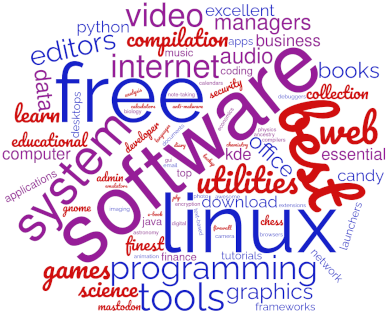# 15 Best Free and Open Source Linux Computer Algebra Systems

A computer algebra system (CAS) is mathematical software that can manipulate mathematical formulae in a way similar to the traditional manual computations of mathematicians and scientists. This type of system supports a wide range of mathematics including linear algebra, calculus, and algebraic and ordinary differential equations.

A CAS offers a rigorous environment for defining and working with structures such as groups, rings, fields, modules, algebras, schemes, curves, graphs, designs, codes and many others.

They have been extensively used in higher education.

The main features of a CAS include:

• Numerical Computations: The software can determine numerical approximations of solutions, derivatives, integrals, differential equations, etc. Solve, manipulate, and plot functions without needing to generate numeric data. Often problems that cannot be solved explicitly can be solved numerically, and often only a numerical answer is sufficient.
• Data Analysis: Having data is not sufficient; we need to extract useful information from it. There are many algorithms designed for data analysis, most of which involve too much work to be done by manual computations. CAS’s put these algorithms in one place, and offer an environment where the algorithms are easy to implement.
• Data Visualization: CAS’s can graph 2D and 3D functions in a variety of ways. They are also designed to graph vector fields and solutions to differential equations.
• Symbolic Computations: Most of the CAS’s can perform symbolic manipulation of expressions: reducing, expanding, simplifying, derivatives, antiderivatives, etc. Unlike numerical computations, which can exhibit floating-point errors, symbolic computations are determined exactly. They can therefore provide the exact answer to an equation (as opposed to a decimal approximation), and they can express results in terms of a wide variety of previously defined functions.

A CAS automates tedious and sometimes difficult algebraic manipulation tasks. The principal difference between a CAS and a traditional calculator is the ability to deal with equations symbolically rather than numerically.

The chart below offers our rating for each software. Some of the software is very specialized, designed to fill a particular niche. This makes comparisons difficult. All of the software is free and open source goodness.Computer Algebra Systems
Scilab Numerical computational package
Maxima System for the manipulation of symbolic and numerical expressions
Octave Powerful programming language with built-in plotting and visualization tools
SageMath Open source alternative to Magma, Maple, Mathematica and Matlab
SymPy Python library for symbolic mathematics
SINGULAR Computer Algebra System for polynomial computations
Macaulay2 Software system for research in algebraic geometry
Cadabra Symbolic computer algebra system for field theory problems
PARI/GP Widely used algebra system designed for fast computations in number theory
GAP System for computational discrete algebra
FriCAS Fork of Axiom
CoCoA System for doing computations in commutative algebra
Mathics General-purpose CAS with Mathematica-compatible syntax and functions
FORM Symbolic Manipulation System
Axiom General purpose Computer Algebra systemRead our complete collection of recommended free and open source software. Our curated compilation covers all categories of software. The software collection forms part of our series of informative articles for Linux enthusiasts. There are hundreds of in-depth reviews, open source alternatives to proprietary software from large corporations like Google, Microsoft, Apple, Adobe, IBM, Cisco, Oracle, and Autodesk. There are also fun things to try, hardware, free programming books and tutorials, and much more.